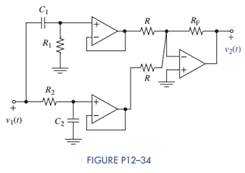### Create an Account

Home / Questions / The circuit in Figure P12 34 produces a bandstop response for a suitable choice of elemen...

# The circuit in Figure P12 34 produces a bandstop response for a suitable choice of element values Find the circuit’s transfer function Identify the elements that control

The circuit in Figure P12–34 produces a bandstop response for a suitable choice of element values.(a) Find the circuit’s transfer function.

(b) Identify the elements that control the two cutoff frequencies. Select the element values so that the cutoff frequencies are 400 krad/s and 4000 krad/s. Use practical element values with resistances greater than 10 kΩ and capacitances less than 1 μF. Design your passband gain to be + 40 dB. After looking at the simulations, can the design specifications be met?

(c) Use MATLAB to plot the Bode magnitude plot for the values you selected.

(d) Simulate your circuit using Multisim and compare the results to the MATLAB output.

May 24 2020 View more View LessSubscribe To Get Solution